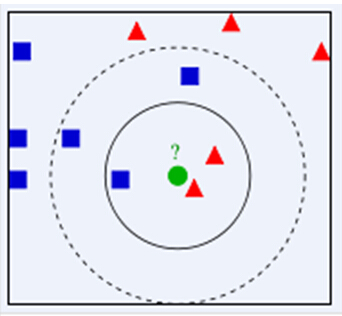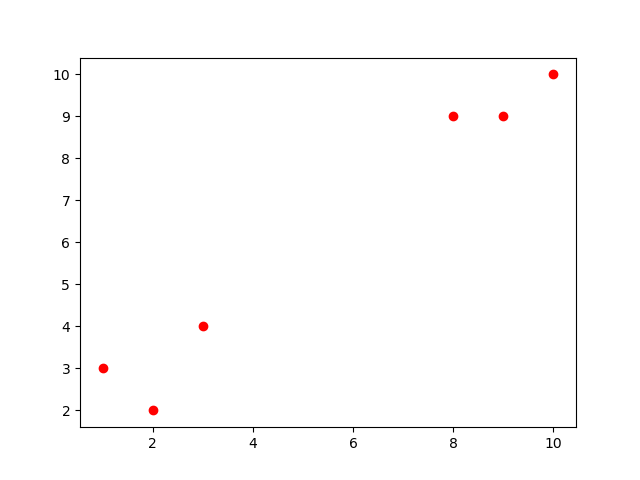# python系列之手写KNN（k-近邻）聚类算法

2019-06-14 19:37 405 查看

KNN（k-Nearest Neighbors）是一种比较基础的机器学习分类算法，该算法的思想是：一个样本与数据集中的k个样本最相似，如果这k个样本中的大多数属于某一个类别，则该样本也属于这个类别。具体案例包括通过动作镜头及接吻镜头的次数对电影的类型进行区分等等，下面会进行详细讲述。

# 1.KNN原理介绍

所谓近朱者赤、近墨者黑，你身边哪种类型的朋友最多，就把你定义为这中类型的人。knn就是利用这一思想，通过测试数据的近邻类别最多的一类作为测试数据的类别。简单的说，knn就是采用测量不同特征之间的距离方法进行分类。
这里利用已经比较经典的图片说明，如下图所示，绿色圆要被决定赋予哪个类，是红色三角形还是蓝色四方形？如果K=3，由于红色三角形是最多的类，因此绿色圆将被赋予红色三角形那个类，如果K=5，由于蓝色四方形是最大的类，因此绿色圆被赋予蓝色四方形类。当然，关于距离的计算存在很多中方式，比如欧式距离、曼哈顿距离等等，这里的代码实现中用的是欧式距离。

优点：
(1) 精度高；
(2) 对异常值不敏感。
缺点：
(1) 计算复杂度高；
(2) 空间复杂度高。
适用数据范围：
数值型和标称型

# 3.具体步骤如下：

(1) 计算测试数据与各训练数据之间的距离；
(2) 对距离进行升序排列；
(3) 选取距离最小的K个点；
(4) 计算前K个点各自类别的出现频率；
(5) 返回前K个点中出现频率最高的类别作为测试数据的预测分类。

# 4.python实现：

```#pyhton3.6
import pandas as pd
import numpy as np
##算法编写
def whlknn_one(data_train,data_test,k):
#1 查看数据规模
trainCol=data_train.shape#列数
trainRow=data_train.shape#行数-对比数据个数
testCol=data_test.shape  #列数

#2 计算测试数据到训练数据各点的距离
diff_i=*trainRow
for i in range(0,trainRow):
diff_j=*testCol
for j in range(0,testCol):
diff_j[j]=(data_test.iloc[0,j]-data_train.iloc[i,j])**2
diff_i[i]=sum(diff_j)**0.5

#2 排序并取前k个距离最小的类别,k值是否超过了训练数据的行数，超过则取最大行数
if k<=trainRow:
num = np.argsort(diff_i)[:k]
else:
num = np.argsort(diff_i)[:trainRow]

#3 取出前k个距离最小的训练集中所对应的类别
typeTot=data_train.iloc[num,trainCol-1]

#4 取出最多的一类作为测试集的分类结果
count={}
for tp in typeTot:
count.setdefault(tp,0)
count[tp]=count[tp]+1

#5 取出最多的类作为测试数据的类别
result=max(count,key=count.get)
return result

#多行测试数据循环调用whlknn
def whlknn(data_train,data_test,k):
rowTest=data_test.shape  #测试数据列数
tsetType=[None]*rowTest
for s in range(rowTest):
tsetType[s]=whlknn_one(data_train,data_test[s:s+1],k)
return tsetType```

接下来对算法进行测试：```##代码测试
##训练集
data_train1=pd.DataFrame(np.arange(10).reshape((5,2)),columns=['x1','x2'])
data_train1.loc[:,'y']=['a','a','a','b','b']
#print(data_train)
##测试集
a1=[1,2]
a2=[8,9]
b1=['x1','x2']
data_test1=pd.DataFrame(data=[a1,a2],columns=b1)
#print(data_test)

ss=whlknn(data_train1,data_test1,3)
print('测试类别为：',ss)
#输出结果为：

结果输出，a1、a2分别为a、b类。

欢迎交流哟~